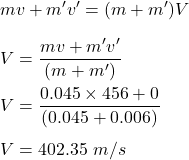## 7. A 0.045-kg bullet traveling at a speed of 456 m/s is fired vertically into a sponge with a mass of 0.0060kg that is initially at rest. As

Question

7. A 0.045-kg bullet traveling at a speed of 456 m/s is fired vertically into a sponge with a mass of 0.0060kg that is initially at rest. Assuming that the bullet is embedded in the sponge, how high would the combined bullet and sponge rise after the perfectly inelastic collision?

in progress 0
6 months 2021-07-27T04:10:40+00:00 2 Answers 16 views 0

Explanation:

To find the velocity of combined body we shall apply conservation of momentum

initial momentum = .045 x 456 = 20.52 kg m /s

mass of combined body = .045 +.006

= .051 kg

If v be the velocity of combined mass

.051 v = 20.52

v = 20.52 / .051

= 402.35 m/s

If h be the heights up to which combined mass go with deceleration g

v² = 2gh

h = v² / 2g

= 402.35² / 2 x 9.8

= 8259.46 m

Combined velocity is 402.35 m/s.

Explanation:

Given that,

Mass of the bullet, m = 0.045 kg

Initial speed of the bullet, v = 456 m/s

Mass of the sponge, m’ = 0.006 kg

Initial speed of the sponge is 0 as it was at rest. The momentum remains conserved in this case. The bullet is embedded in the sponge, it is a case of inelastic collision. So,So, the combined bullet and sponge velocity after the perfectly inelastic collision is 402.35 m/s.